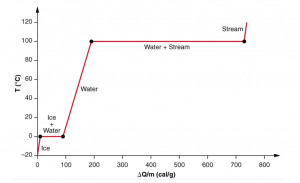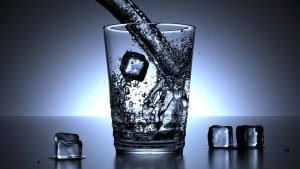# Latent Heat Of Vaporization And Fusion

## What is Latent Heat?

There comes a point while heating a cube of ice when there is no change in temperature in spite of continuous heating. There will be absolutely no rise in the temperature till the ice cube has melted entirely. The amount of heat required to change the state of a unit mass of a substance from solid to liquid or from liquid to vapour without changing its temperature is known as the latent heat.

In short, latent heat is defined as:

The amount of heat that changes the state of the material without changing its temperature.

The heat is either absorbed or released when a body undergoes a constant-temperature process. In this article, we will be discussing the latent heat of vaporization and latent heat of fusion and their characteristics in detail.

## Types of Latent Heat

Based on the different phases of the substance, there are two types of latent heats as follows:

### Latent Heat of Fusion

We can define the Latent Heat of Fusion as the amount of heat required to change a unit mass from solid to liquid at a constant temperature. In general, it is the heat required to melt the substance from solid or the amount of heat to be removed from a unit substance to freeze it from liquid to solid.

The latent heat of fusion of water is:

 $L_{f}$ = $3.33\times 10^{5}Jkg^{-1}$

### Latent Heat of Vapourisation

Latent Heat of Vapourisation is defined as the amount of heat required to change the unit mass of liquid at boiling point into vapour under constant temperature. In general, it is the heat required to vaporise a liquid or the amount of heat to be removed from gases to condense it into a liquid.

The latent heat of vaporization of water is:

 $L_{f}$ = $22.6\times 10^{5}Jkg^{-1}$

### Latent heat of fusion and vapourisation of ice, water and various other substances:

In the table below, the latent heat of fusion of ice and various other substances along with their latent heat of vapourisation is given below:

 Substance Specific latent heat of fusion kJ.kg-1 Specific latent heat of vaporisation kJ.kg-1 Water 334 2258 Oxygen 14 213 Hydrogen 60 449 Ethanol 109 838 Chloroform 74 254

Latent Heat of Vaporization is 7.5 times as large as the Latent Heat of fusion i.e. the energy required to melt something is considerably lower than the energy required to vaporize something. This is the reason why a burn caused by steam at 1000C will be a lot more severe than a burn caused by water at 1000C. The graph below shows the two state changes of water and their corresponding temperature changes.### What causes the temporary stagnancy in temperature?

If we continue heating the ice cube, then we reach a temperature where the water changes states from liquid to gas; 1000C. At this temperature, water starts escaping the surface in the form of water vapour. From the instant the water touches 1000C and starts boiling till the time the entire water sample is boiled off, the water’s temperature remains constant. Now we see a pattern, don’t we? This stagnancy in temperature seems to occur along with the change of state. Why so?

To understand this behaviour, we will have to examine the molecular behaviour. In case of ice, the molecules are packed together and are mostly immovable. They still do vibrate in their position, but they don’t flow from place to place. This represents the lowest energy state of water. Now when you heat ice cubes, you are supplying energy in the form of heat and this energy increases the vibration of the molecules in the ice.On continued heating, the water molecules gain enough energy to break the hydrogen bonds of the H2O which gives ice its rigidity. So the thing is, water starts melting at 00C but to break these hydrogen bonds, it needs more heat. This heat is known as the Latent heat.

## Latent Heat Formula

If the amount of latent heat required to change the state of a substance of mass ‘m’ is ‘Q’ then the latent heat formula is:

 $L$ = $\frac{Q}{m}$

After the ice has all melted, the temperature of the water begins to rise again till it reaches 1000C. If Ice is the lowest energy state of water, gas is the other extreme; gas molecules have the most energy and are constantly whizzing around. This energy to whizz around comes from the heat we supply to it. Remember that water exists as a liquid due to intermolecular forces of attraction which needs to be overcome in order to turn it into gas.

Once it reaches 1000C, the energy supplied is used by the water molecules to break its bonds with other molecules and run away. This energy doesn’t show up as a temperature change as in the case of melting but is used by the molecules to break the bonds and enter a higher energy state i.e. the gaseous state.

## Examples of Latent Heat

• Latent heat intensifies hurricanes. Air heats as it passes warm water and picks up water vapour. As the vapour condenses to form clouds, latent heat liberated into the atmosphere. The liberated heat warms the air, causing instability and helps clouds rise resulting in the storm to intensify.
• Boiling water on a stove releases an enormous amount of energy because water possesses a high latent heat of vaporization.
• Similarly, water has high latent of fusion hence a considerable amount of energy must be absorbed to convert liquid water to ice in a freeze.If you wish to learn more physics concepts with the help of interactive video lessons, download BYJU’S – The Learning App.